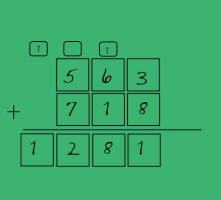# Math SMARTBoard Files

###Adding Three Addends Math GamePrintout for classroom game Adding Three Addends Game###Fact Family GeneratorRoll the dice to create fact families.
Printout for Fact Family Generator###Addition and Subtraction With RegroupingStudents use number generator to create and solve problems.

###Addition TemplateTemplate to write and solve addition problems.

###Subtraction TemplateTemplate to write and solve subtraction problems.

###Morning CalendarCalendar for use with morning meeting.

###Calendar Time TemplateBlank calendar template.

###Time to Half-Hour ClockClock is shaded into two halves to explain half-hour time.

###Telling TimeStudents can manipulate the hands on the clock or write the digital time.

###Write the TimeStudents write the time shown on the clock by the teacher.

###Counting ChangeStudents count coins.

###Place Value to 100Generate how many hundreds, tens, and ones needed and then show the number with blocks and notation.

###Two Digit Addition With Regrouping Ones PlaceStudents solve problem and choose correct answer.

Two Digit Addition With Regrouping 2 Ones PlaceStudents solve problem and choose correct answer.

Two Digit Addition With Regrouping 3 Ones PlaceStudents solve problem and choose correct answer.

###Two Digit Addition With Regrouping Tens PlaceStudents solve problem and choose correct answer.

Two Digit Addition With Regrouping Tens Place 2Students solve problem and choose correct answer.

Two Digit Addition With Regrouping Tens Place 3Students solve problem and choose correct answer.

###Place Value to 100 GeneratorGenerate a three digit number and show the number using blocks, standard notation, and expanded notation.

###Place Value to 100Generate a two digit number and show the number using blocks, standard notation, and expanded notation.

###Skip Counting PracticePractice skip counting with the hundred chart.

###Doubles Plus OneIntroduces doubles plus one concept to students.